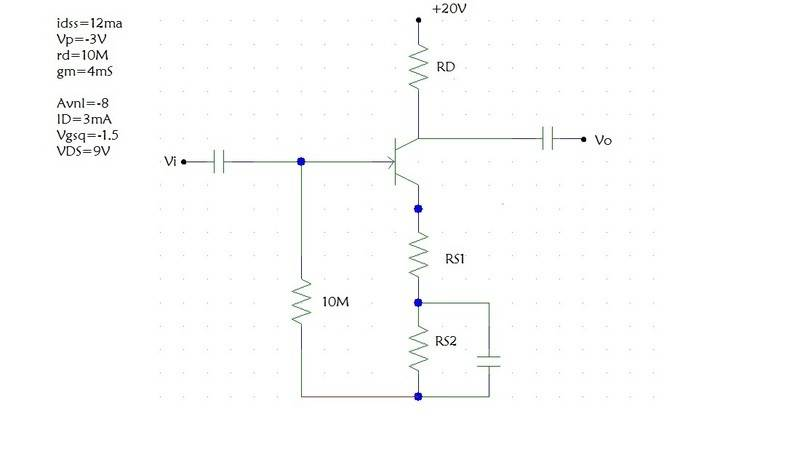# Self Bias Transistor Design Problem

## Homework Statement

I have to solve for RD, RS1, and RS2.## Homework Equations

AVNL=-mg(Rd//rd)
Vdd-Id(Rd+RStotal)=0
Bias Line Eq: Vg-Vgsq-Idq(RStotal)

## The Attempt at a Solution

I have solved for RD by using the AVNL equation and the given value of -8 for AVNL.
I found RD to be 2000 ohms.

I cannot figure out how to get Rs1 and Rs2.

uart
Science Advisor

## The Attempt at a Solution

I have solved for RD by using the AVNL equation and the given value of -8 for AVNL.
I found RD to be 2000 ohms.

That's not correct. The voltage gain can only be approximated as (-gm Rd) if there is no un-bypassed source resistance (RS1).

Start by using the knowledge of Vgs (quiescent) to find the total source resistance (RS1+RS2), and then use the knowledge of Vds (quiescent) to find the value of RD.

Finally you need to derive (or look up) a correct expression for the voltage gain to solve for RS1.